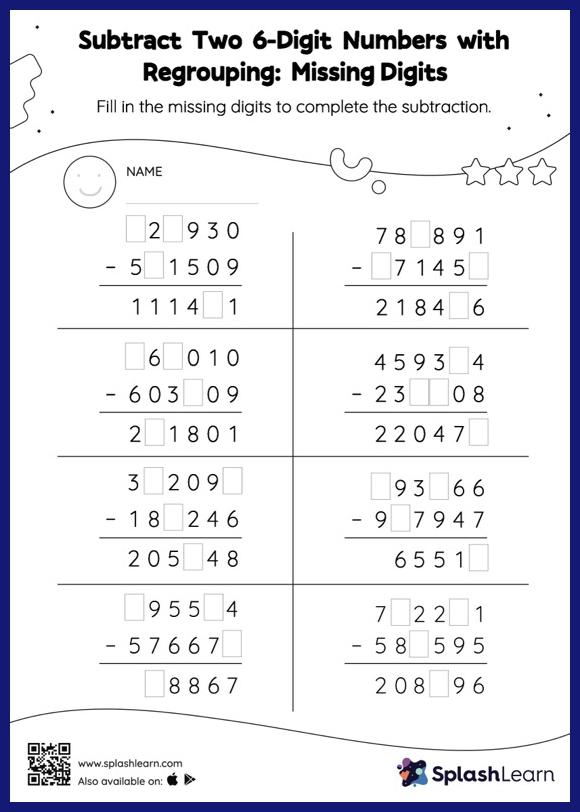# Subtract Two 6-Digit Numbers with Regrouping: Missing Digits Worksheet

Home > Subtract Two 6-Digit Numbers with Regrouping: Missing DigitsStudents use the count back strategy or the relationship between addition and subtraction to find the missing number in this worksheet. They also need to regroup numbers to get to the answer in subtract two 6-digit numbers with regrouping worksheet. As the worksheet uses the column method, it is helpful in getting students toward higher accuracy, especially with bigger numbers and in scenarios where regrouping is required.